Get inspired by the success stories of our students in IIT JAM MS, ISI  MStat, CMI MSc DS.  Learn More

# Let's Permute | ISI MStat 2018 PSB Problem 3This problem is an easy application of the basic algorithmic ideas to approach a combinatorics problem using permutation and combination and basic counting principles. Enjoy this problem 3 from ISI MStat 2018 PSB.

## Problem

Consider all permutations of the integers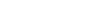. In how many
of these permutations will the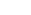number be the minimum of the
first 25 numbers and thenumber be the minimum of the first 50
numbers?

## Solution

Whenever you are counting, think in terms of algorithms or steps in which you will do the task given in the problem and count each of the steps. Now the conjunction in between the steps ( and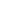, or) will show you the way.

#### Steps

Select the first 50 numbers. Fix the minimum element. Out of the 49 other elements, select the first 25 numbers. Fix the minimum element of these 25 numbers. Then permute the rest.

AND for each of these selections of two sets (Multiplication Principle),

#### Step 2

Once you select the three sets, the minimum of each of these sets is already fixed. So, for each of these sets, you have to permute the other first 24 numbers (1 - 24) and the next 24 numbers (from 26 - 49) and the other 50 numbers (50 - 100).

You can do that in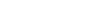ways.

Therefore, you can do the whole task in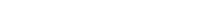ways.

## Food for Thought

• Do the problem for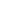numbers.
• Do the problem if you want to fix the minimum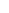numbers in each set out ofnumbers.
• Do the problem if you want to fix the minimum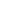numbers in each set out ofnumbers. Does it match your expectation?
• Generalize and Degeneralize and make your own problem out of this. Comment it below. We will update it here if we think the problem is appropriate.

This problem is an easy application of the basic algorithmic ideas to approach a combinatorics problem using permutation and combination and basic counting principles. Enjoy this problem 3 from ISI MStat 2018 PSB.

## Problem

Consider all permutations of the integers. In how many
of these permutations will thenumber be the minimum of the
first 25 numbers and thenumber be the minimum of the first 50
numbers?

## Solution

Whenever you are counting, think in terms of algorithms or steps in which you will do the task given in the problem and count each of the steps. Now the conjunction in between the steps ( and, or) will show you the way.

#### Steps

Select the first 50 numbers. Fix the minimum element. Out of the 49 other elements, select the first 25 numbers. Fix the minimum element of these 25 numbers. Then permute the rest.

AND for each of these selections of two sets (Multiplication Principle),

#### Step 2

Once you select the three sets, the minimum of each of these sets is already fixed. So, for each of these sets, you have to permute the other first 24 numbers (1 - 24) and the next 24 numbers (from 26 - 49) and the other 50 numbers (50 - 100).

You can do that inways.

Therefore, you can do the whole task inways.

## Food for Thought

• Do the problem fornumbers.
• Do the problem if you want to fix the minimumnumbers in each set out ofnumbers.
• Do the problem if you want to fix the minimumnumbers in each set out ofnumbers. Does it match your expectation?
• Generalize and Degeneralize and make your own problem out of this. Comment it below. We will update it here if we think the problem is appropriate.

This site uses Akismet to reduce spam. Learn how your comment data is processed.

### 4 comments on “Let's Permute | ISI MStat 2018 PSB Problem 3”

1.Uttaran Chatterjee says:

Shouldn't it be choosing 50 numbers from100 in 100C50 ways, and these 50 numbers can be ordered in 1 way, so say the first 50 numbers are arranged in decreasing order, now fixing the position of the 25th and 50th number the first 24 numbers can be arranged in 24! Ways, and the numbers between 25th and 50th can also be arranged in 24! , So it becomes 100C50*24!*24! ways total number of possibilities.

1.Srijit Mukherjee says:

Yeah, you are right. I will make the changes.

2.Soham Ghosh says:

I feel there is a snag somewhere. Let P be the random permutation.See that there are no restrictions in choosing P(51),..P(100) .The 50th element would have to be the minimum of the remaining 50. Again there are no restrictions in choosing P(49),..,P(26). The 25th element should be the minimum among the rest and then again no restrictions in choosing P(24),..P(1). So, the total number of ways=100*99*...*51*49*..26*24*..*1
=100! /(25*50)

1.Srijit Mukherjee says:

Yeah, you are right. I will make the changes.

### Knowledge Partner